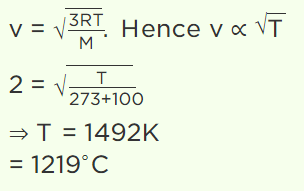## Kinetic Theory Questions and Answers Part-11

1. The absolute temperature of a gas is determined by
a) The average momentum of the molecules
b) The velocity of sound in the gas
c) The number of molecules in the gas
d) The mean square velocity of the molecules

Explanation: The absolute temperature of a gas is determined by the mean square velocity of the molecules

2. The root mean square velocity of the molecules in a sample of helium is 5/7th that of the molecules in a sample of hydrogen. If the temperature of hydrogen sample is 0°C, then the temperature of the helium sample is about
a) 0°C
b) 0 K
c) 273°C
d) 100°C

Explanation:3. At what temperature is the root mean square velocity of gaseous hydrogen molecules is equal to that of oxygen molecules at 47°C
a) 20 K
b) 80 K
c) – 73 K
d) 3 K

Explanation:4. For a gas, the r.m.s. speed at 800 K is
a) Four times the value at 200 K
b) Half the value at 200 K
c) Twice the value at 200 K
d) Same as at 200 K

Explanation: For a gas, the r.m.s. speed at 800 K is twice the value at 200 K

5. The root mean square velocity of a gas molecule of mass m at a given temperature is proportional to
a) $m^{0}$
b) m
c) $\sqrt{m}$
d) $\frac{1}{\sqrt{m}}$

Explanation: The root mean square velocity of a gas molecule of mass m at a given temperature is proportional to $\frac{1}{\sqrt{m}}$

6. A sample of gas is at 0°C. To what temperature it must be raised in order to double the r.m.s. speed of the molecule
a) 270°C
b) 819°C
c) 1090°C
d) 100°C

Explanation:7. A gas is allowed to expand isothermally. The root mean square velocity of the molecules
a) Will increase
b) Will decrease
c) Will remain unchanged
d) Depends on the other factors

Explanation: A gas is allowed to expand isothermally. The root mean square velocity of the molecules will remain unchanged

8. The temperature at which the root mean square velocity of a molecule will be doubled than at 100°C
a) 1219°C
b) 1492°C
c) 400°C
d) 400 K

Explanation:9. At room temperature, the r.m.s. speed of the molecules of certain diatomic gas is found to be 1930 m/s. The gas is
a) $H_{2}$
b) $F_{2}$
c) $O_{2}$
d) $Cl_{2}$